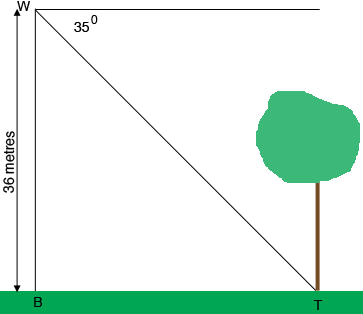SEARCH HOMEMath Central Quandaries & QueriesQuestion from Ranger_minor, a student: A woman of height 1.4 metres standing on the top of a building 34.6 metres high views a tree some distance away. she observes that the angle of depression of the bottom of the tree is 35 degrees and the angle of depression of the top of the tree is 29 degrees. assume that the building and the tree are on level ground : 1). calculate the distance of the woman from the top of the tree measured along her line of sight.Hi,

I can help get you started.

In my diagram $B$ is the base of the building, $T$ is the base of the tree and $W$ is the woman's eyes. Since the angle of depression to the base of the tree is $35^o$ the measure of the angle $TWB$ is $55^o.$Use some trigonometry to find the distance from $B$ to $T.$

PennyMath Central is supported by the University of Regina and The Pacific Institute for the Mathematical Sciences.